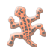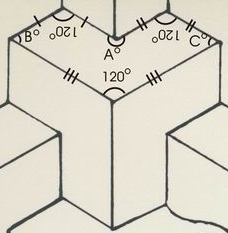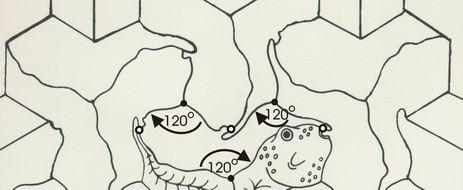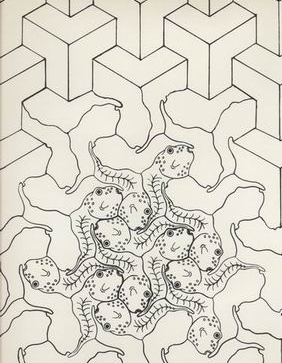back to history & resources# A Formula for Irregular Tessellating Hexagons

by Sam Savage

I came up with the following tessellation formula in 1976. Later I discovered that it had originally been proven in around 1916, so I had come within 60 years of the forefront of mathematics.

Start with three straight lines of different lengths, making sure that the longest line is not longer than the sum of the two shorter ones.

 Make two copies of each line joined by a 120 degree angle, then hook the three pairs of lines together. The resulting hexagon will tessellate. In the example here, the two shorter lines are equal in length and sum exactly to the length of the longer line.To see why the resulting figure tessellates, imagine rotating the shape about any of its 120 degree vertices. Since 3 times 120 degrees equals 360 degrees, exactly three such shapes will fill up all the space around any such vertex.

Now how about the other three vertices? You will see that the total angle around each of the non-120 degree vertices is equal to A+B+C. But the internal angles of any hexagon sum to 720 degrees, and since we have already used up 360 degrees with the three 120 degree angles, A+B+C must also equal 360 degrees. Hence the space is filled at the other three vertices.

Once you have a tessellating hexagon, you can replace the straight lines with curves that are duplicated and rotated 120 degrees.This formula forms the basis of the SHMUZZLE (tm) Puzzle as well as From Polygons to Pollywogs, a silkscreen I did in the early 1980’s.